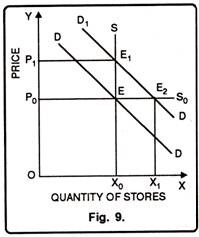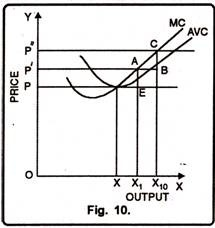# The Concept of Quasi-Rent (With Diagram)

The concept of quasi-rent was first of all introduced by Dr. Marshall.

According to him, “The term quasi rent will be used in the present volume as income derived from machines and other appliances of production made by man.”Basically”, the term “Quasi” has been derived from Latin word, meaning ‘as if. Therefore, it refers to “as if rent”.

Like Ricardo, Marshall also believed that land earns rent because its supply is scare. In the short-run, the supply of machines and other man-made goods is also inelastic. Suppose there is an increased demand for machines.

In the short-run, the supply of neither land nor machines can be increased to meet the changes in demand. Thus, in the short run, whenever there is a rise in demand for machines and other man-made appliances, they will earn an income which is something similar to rent. This surplus income which is earned by machines in the short run and which will disappear in the long run has been described as quasi-rent.

“The additional payment for those agents of production the supply of which though alterable in a long period, is fixed in short period is technically known as quasi-rent”. Silverman

Marshall has tried to explain the concept of Quasi Rent through the example of “Parable of meter of stone”. This shows how the inelastic supply of the factors in the short run earns rent for them.

### Assumptions:

1. It is assumed that some stones are gifted by nature only to a particular place.

2. It is also supposed that supply of these stones in the short period cannot be changed.

#### Quasi Rent from Inelastic Supply of Factors:

In order to know how the inelastic supply of the factors earns rent in the short-run, let us explain with the help of a diagram.

In fig. 9, X0S is the short run supply curve and DD is the demand curve of stones. In the short run, since, supply is fixed at OX0, the equilibrium per stone price will be OP0. But, in the long run, and supply becomes perfectly elastic as P0S0 shown in the fig. The demand curve D1D1 intersects the supply curve at point E1 and quasi rent is OX0 E1P1. But in the long run the supply becomes equal to demand. The new demand curve cuts the elastic supply at E2, so, no rent is earned. The price which prevails is equal to the cost of finding stones. Thus, no surplus is earned.#### Quasi Rent as a Surplus over Variable Cost:

Marshall defined quasi-rent as the excess of total revenue over the total variable costs. In the short time, there are two types of factors of production viz; fixed factors and variable factors.

Fixed factors in the short period remain constant and the quantity of variable factors depends on the size of the production and there exists a shut down point in the short run.

The price must cover the variable costs. But in the long period the price covers both the fixed as well as variable costs.

Therefore, quasi rent can be expressed as:

Quasi Rent = Total Revenue — Total Variable Costs.

The quasi rent can be illustrated through Figure 10 where output has been shown on OX-axis and price on OY-axis. MC and AVC are marginal cost and average variable cost curves respectively.

At equilibrium price OP output is OX. Since price is equal to AVC, the firm is getting no quasi-rent. Now if the price rises to OP’, the firm will produce OX1 output which is more than AVC i.e. by AE, thus, it will earn quasi-rent AX1 — EX1 = AE. The total quasi-rent will be AEPP’. In the same way if price rises to OP”, quasi-rent will also increase to the extent of CBP’P”.### Quasi Rent and Interest:

Quasi rent should be distinguished from interest. Interest is a return from capital. On the other hand, quasi rent is the return from the specialized or sunk capital i.e., from old investments of capital. In the words of Marshall, “That which is rightly regarded as interest on ‘free’ or ‘floating’ capital or on new investments of capital, is more properly treated as a sort of rent-a quasi rent on old investments of capital. And there is no sharp line of division between floating capital and that which has been sunk from a special branch of production, nor between new and old investments of capital; each group shades into the other gradually.” Rent is thus ‘a leading species of a large genus’.

### Rent Element in Other Factors:

#### (i) Quasi Rent and Profits:

We know all the entrepreneurs are not equally efficient. At one extreme, there are those who are just making profit i.e., low profits. Another extreme are those who enjoy high profits due to higher efficiency. They enjoy higher profits which are similar to the surplus enjoyed by superior land. There is thus the rent element in profits. Profits have been called rent of ability.

#### (ii) Rent Element in Wages: